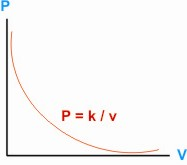HOME MATH DICTIONARY DOWNLOAD FEEDBACK DISCLAIMER
 Question: What is meant by Varies Inversely As ? Answer: When two variables x and y are related by an equation of the form y = k/x, where k is a constant, then y varies inversely as x. For example, the pressure P of a gas at constant temperature varies inversely as its volume V. P = k/v.